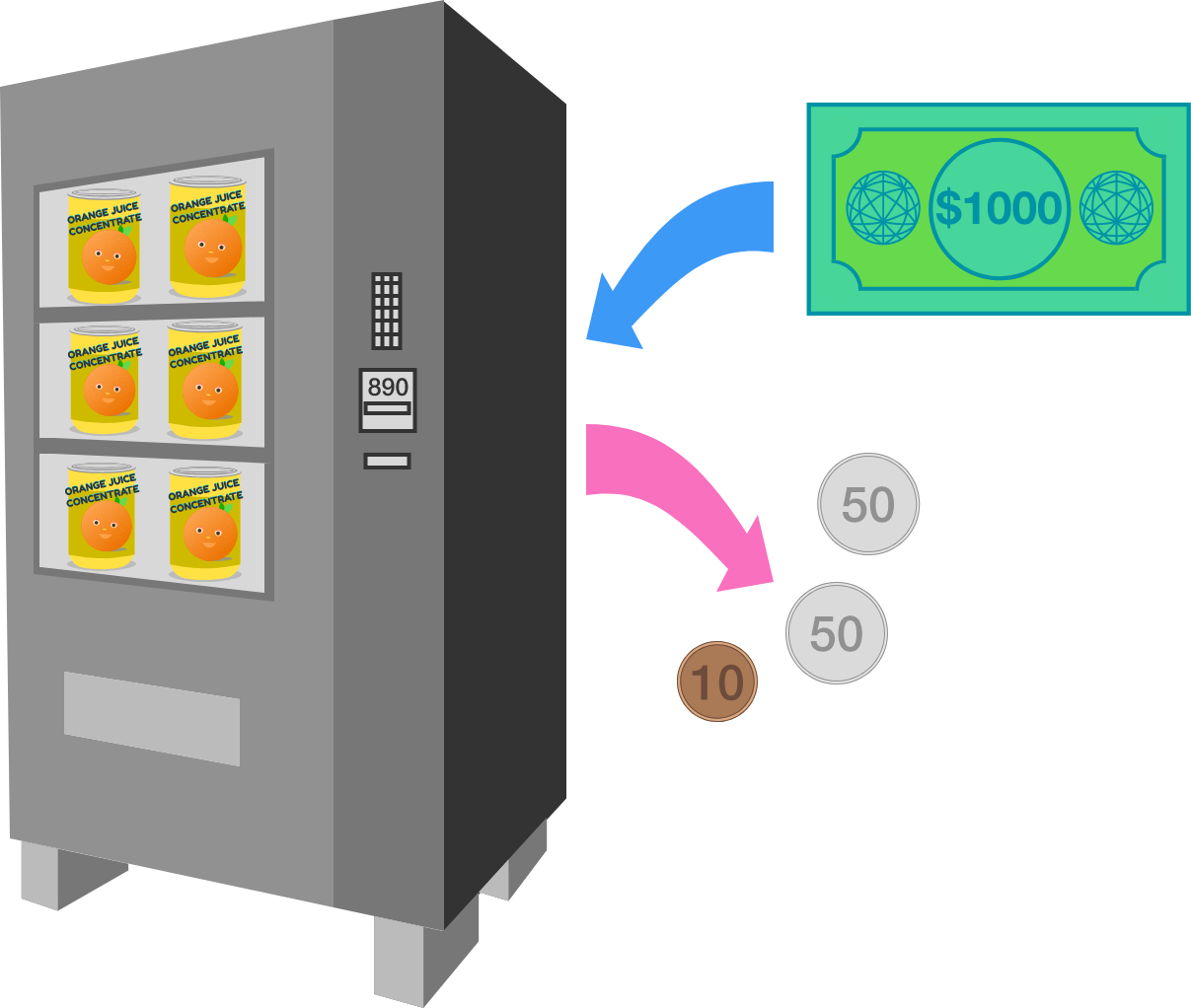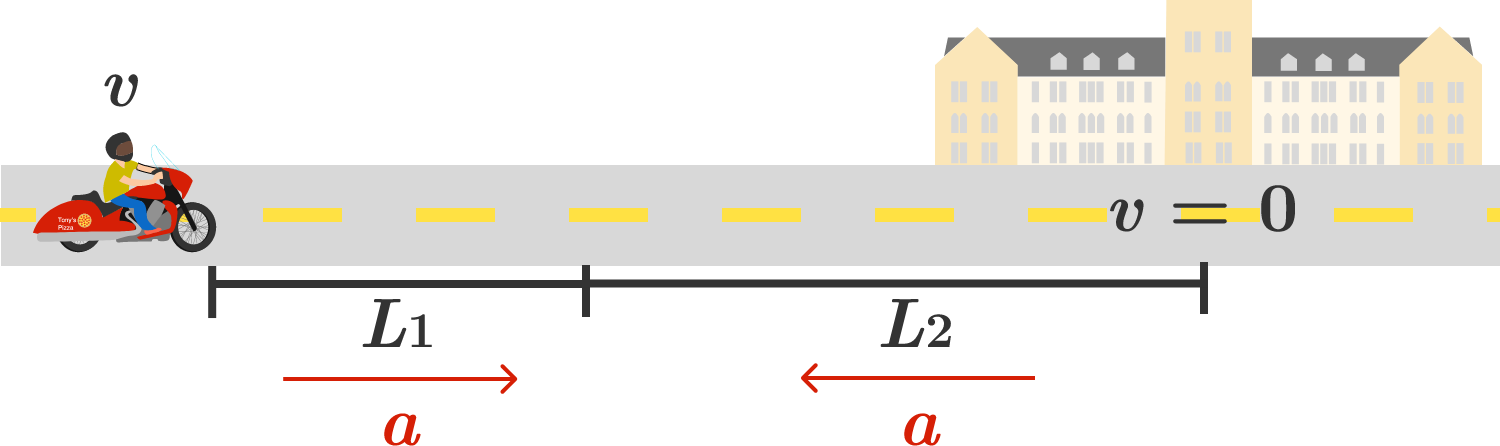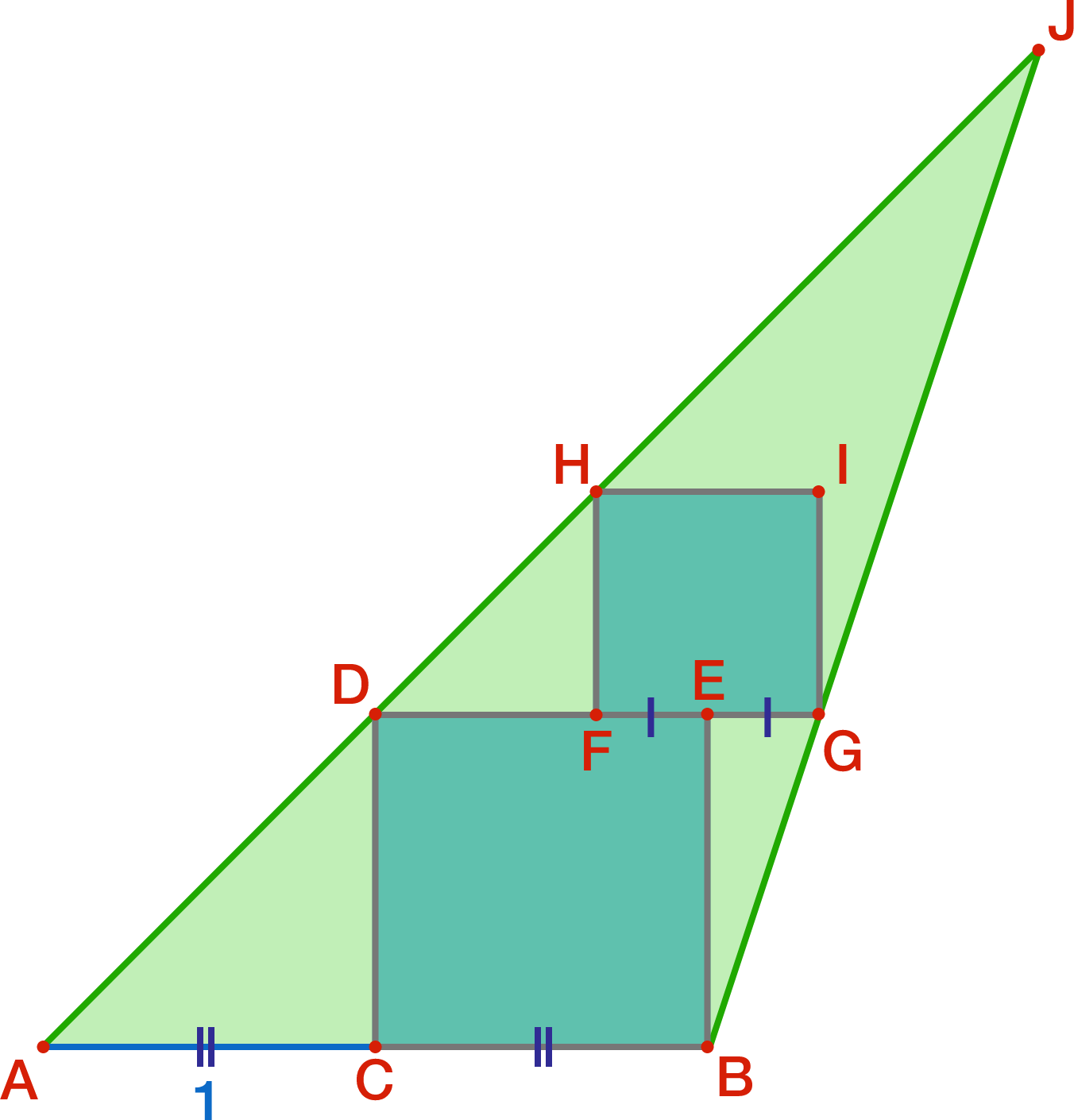# Problems of the Week

Contribute a problem

# 2017-03-06 Intermediate

Is ${\color{#E81990}1}{\color{grey}{2}}{\color{#20A900}{3}}{\color{#3D99F6}{4}}{\color{#D61F06}555}{\color{#3D99F6}{4}}{\color{#20A900}{3}}{\color{grey}{2}}{\color{#E81990}1}$ prime?

Hint: You may use the fact that $111111 \times 111111 = {\color{#E81990}1}{\color{grey}{2}}{\color{#20A900}{3}}{\color{#3D99F6}{4}}{\color{#D61F06}5}6{\color{#D61F06}5}{\color{#3D99F6}{4}}{\color{#20A900}{3}}{\color{grey}{2}}{\color{#E81990}1}$. This is similar to, but not identical to the above number, due to the middle $6$.

A vending machine is designed to return change in the following way:

Keep returning the coin with the largest denomination smaller than or equal to the remaining amount until all of the change has been returned.However, this approach doesn't always yield the least number of coins. With the denominations 10, 40, and 50 cents, which of the following changes (in cents) could be returned using fewer coins than what the algorithm requires?

As an explicit example of the algorithm, when required to produce a 110 cents change, the vending machine would return the coins 50, 50, 10 cents, in that order.Drake is driving a bike on a straight road to his college at a speed of $v$. He realizes that he might be late for his exam.

To rush, he accelerates his bike at a constant acceleration of $a$ for a distance of $L_1$. Then, for the remaining distance $L_2$, he slows down at a constant retardation of $a$ to just reach his college.

Given that $L_2 = k L_1,$ what is the maximum velocity attained during his travel?In the diagram, $AC=1$, where $C$ is the midpoint of side $AB$ of triangle $ABJ$. Square $BCDE$ is drawn, where $D$ lies on side $AJ$ and $E$ is in the interior of the triangle. Square $FGIH$ is drawn, where $G$ lies on side $BJ$, $H$ lies on side $AJ$, $I$ is in the interior of the square, and $E$ is the midpoint of $FG$.

Find the area of triangle $ABJ$.

A bowl contains $N$ strands of noodles. You reach into the bowl and grab two free ends at random and attach them. You do this $N$ times until there are no free ends left.

What is the minimum $N$ such that the expected number of loops generated by the $N$ steps described above exceeds 3?

×